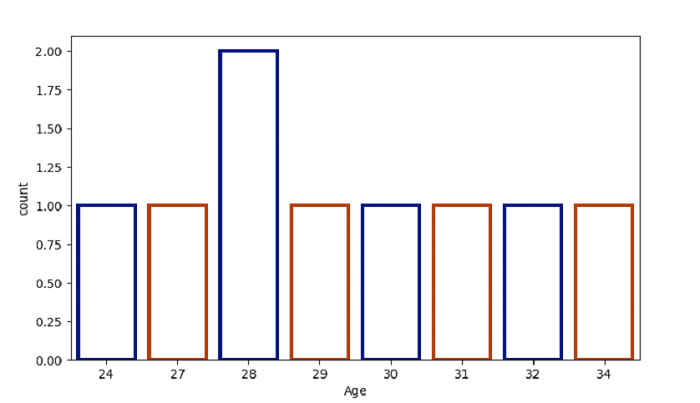# Python Pandas - Create a Count Plot and style the bars with Seaborn

Count Plot in Seaborn is used to display the counts of observations in each categorical bin using bars. The seaborn.countplot() is used for this. Style the bars using the facecolor, linewidth and edgecolor parameters.

Let’s say the following is our dataset in the form of a CSV file − Cricketers.csv

At first, import the required libraries −

import seaborn as sb
import pandas as pd
import matplotlib.pyplot as plt

Load data from a CSV file into a Pandas DataFrame −

dataFrame = pd.read_csv("C:\Users\amit_\Desktop\Cricketers.csv")


Style and design the bars using the facecolor, linewidth and edgecolor parameters −

sb.countplot(dataFrame["Age"], facecolor=(0, 0.0, 0, 0),linewidth=3,edgecolor=sb.color_palette("dark", 2))

## Example

Following is the code −

import seaborn as sb
import pandas as pd
import matplotlib.pyplot as plt

# Load data from a CSV file into a Pandas DataFrame

# plotting count plot with Age column
# designing the bars
sb.countplot(dataFrame["Age"], facecolor=(0, 0.0, 0, 0),linewidth=3,edgecolor=sb.color_palette("dark", 2))

# display
plt.show()

## Output

This will produce the following output −